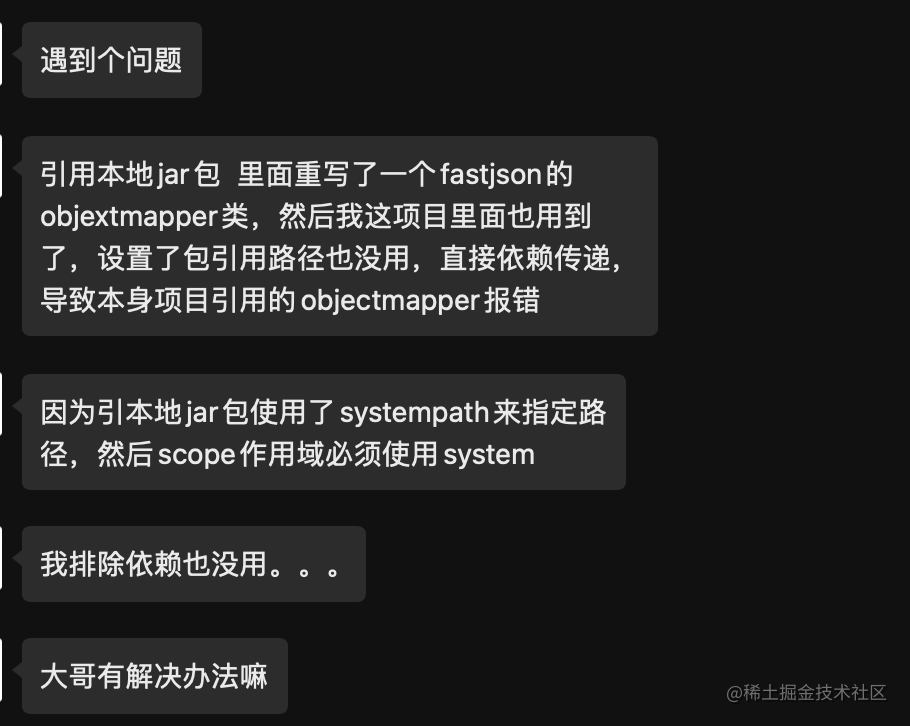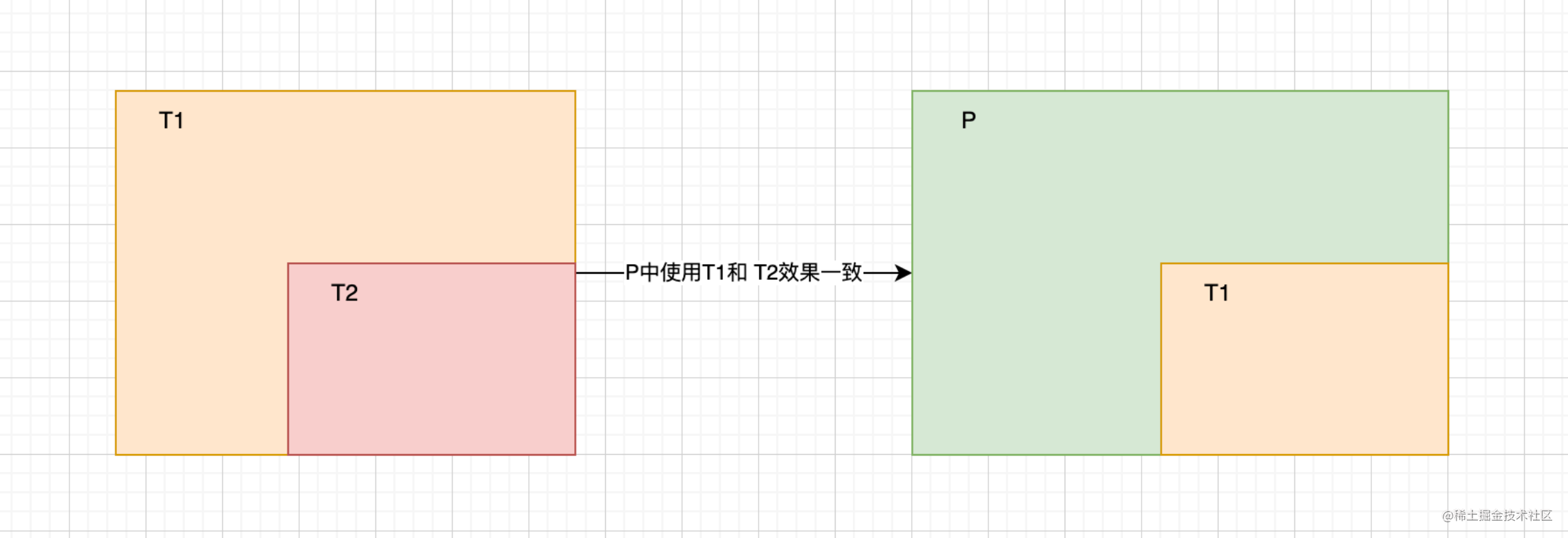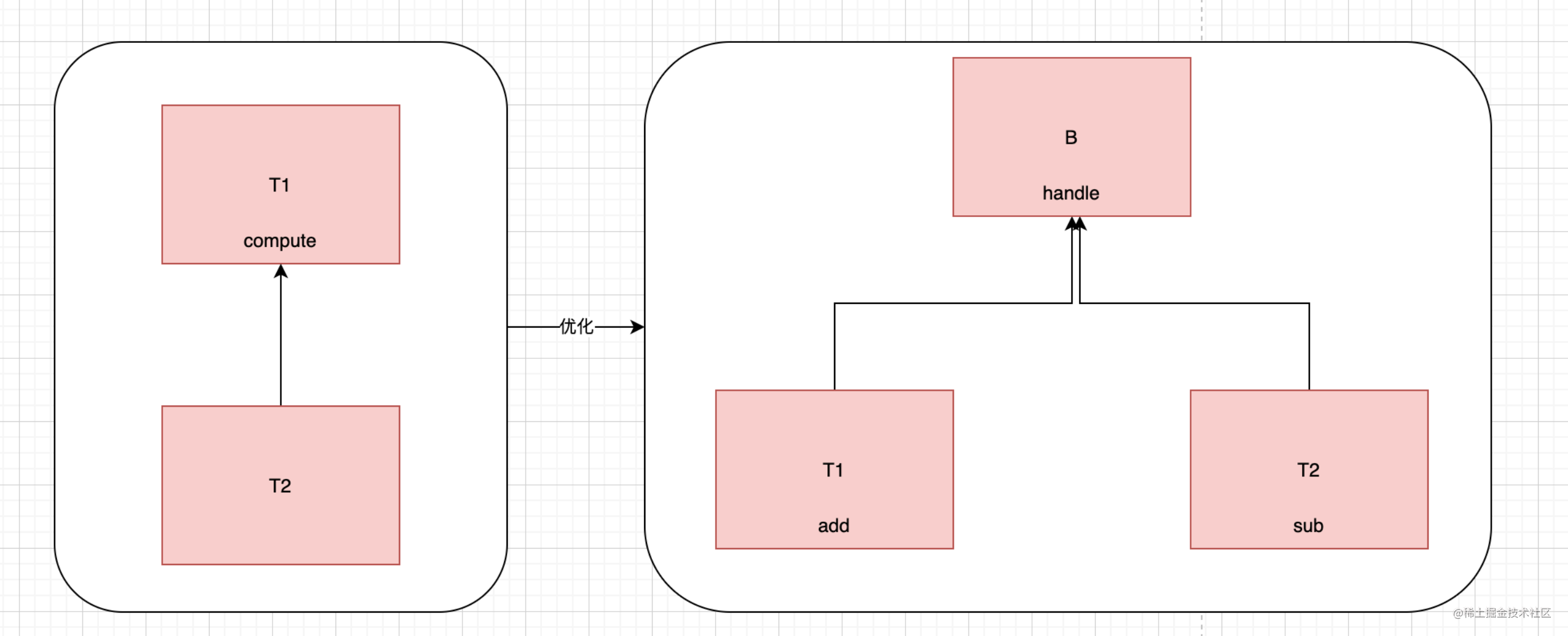# 前言

• 設計模式其實就是前輩在工作中對思想的一種整理。我們都知道Java是面向物件程式設計的。面向物件的三大特點就是繼承，封裝，多型
• 我們今天的主題就是里氏替換原則。他的產生就是解決繼承帶來的問題

# 插曲

• 今天一個好朋友問到我他在使用fastjson但是引入另外一個專案，另外一個專案對內部進行了優化，但是並沒有相容自己的專案。• 針對這種問題我提供了一些自己的見解。我建議他通過雙親機制覆蓋掉其他專案的重寫的部分。但是轉念一想好像又有問題。把別人的覆蓋掉被人的功能可能就會受影響的。

# 迴歸正題

• 後來我們分析了下引入包的修改處，主要是進行的抽象。其他子類通過繼承的方式向fastjson提供小部分的功能。這個被繼承的父類負責整合功能。
• 繼承的主要特點就是設定規則和契約。雖然他並不會強制要求子類進行全部實現。但是他或多或少的要求不能輕易的改動。因為父類的正題規劃而子類只是實現一小部分功能。為什麼上面的問題我們不能直接進行子類的定義擴充套件呢。因為fastjson是別人封裝好的。裡面並沒有提供向外註冊的功能所以我們即使繼承了父類也無法將子類註冊上去。這個問題涉及到fastjson。這裡不做展開。我們主題還是迴歸里氏替換上面。
• 不僅子類受父類的限制，因為是繼承父類也會被子類限制。在1.0中父類設計規劃好後需求升級了父類的整體規劃需要改動。這個時候我們就需要考慮到子類的使用場景。不能僅僅將父類的規劃進行升級。這就是兩個物件增加了耦合性
• 那麼關於繼承的使用我們就需要提出一種約定來避免上面問題的產生。這就是里氏替換原則## 里氏替換

• T2繼承T1，在P程式中T2可以完全替代T1即為里氏替換原則。
• 那麼對於T2有需要不能重寫T1中已經實現好的功能。比如T1中compute方法實現加法的功能而T2重寫了compute變成減法的功能。那就不能進行替換了。
• 但是實際開發中我們不可能不重寫父類的方法。如果又想里氏替換又想重寫，那麼我們只能繼續向上再抽離一層出來了。我們只需要程式中使用B即可。這樣B和T1或者說B和T2之間仍然是里氏替換• 通過上面的優化方式來改進，我們就不會發生子類無法替換父類的情況了。因為我們將父類和子類的關係進行了轉移了。

# 常規開發

```public class LiReplace {      public static void main(String[] args) {          Math math = new Math();          System.out.println("3+4="+math.add(3,4));          ZMath zMath = new ZMath();          System.out.println("3+4="+zMath.add(3,4));          System.out.println("13-4="+zMath.sub(13,4));  ​     }  }  class Math{      public Integer add(int a, int b) {          return a+b;     }  }  ​  class ZMath extends Math {      @Override      public Integer add(int a, int b) {          return a-b;     }  ​      public Integer sub(int a, int b) {          return a-b;     }  }```

• 如果我們必須重寫那就衝破了里氏替換原則了。里氏替換的衝破影響的就是使用者。對於使用者來說我在使用子類替換父類的時候居然達不到父類的功效。最明顯的是現在我有一個抽象類並有一個start方法和待實現的end方法。當我實現了一個子類後。在抽象類中start的方法是將資料寫入到資料庫中。但是因為我實現的這個子類不僅實現了end方法了還實現了start方法了 。那麼這個時候子類必然無法達成父類的功能。對於使用者了來說就無法理解這兩個類之間的關聯與存在的意義了。

```public class LiReplace {      public static void main(String[] args) {          Math math = new Math();          System.out.println("3+4="+math.add(3,4));          ZMath zMath = new ZMath();          System.out.println("3+4="+zMath.addOrSub(3,4));          System.out.println("13-4="+zMath.sub(13,4));  ​     }  }  ​  class Base {      public Integer compute(int a, int b) {          return a*b;     }  }  class Math extends Base{            public Integer add(int a, int b) {          return a+b;     }  }  ​  class ZMath extends Base {            public Integer addOrSub(int a, int b) {          return a-b;     }  ​      public Integer sub(int a, int b) {          return a-b;     }  }```

• 上述就是將父類進行提升。這樣就解決了里氏原則的問題。Math是可以替換Base類.因為並沒有重寫父類的東西。而對於Math和ZMath各自有各自的東西實現，兩者並沒有實際的關聯關係，所以對於使用者來說他們並不會認為兩者有任何的關聯。也就不會造成困擾了。

# 繼續升級

• 仔細觀察下方案一，針對里氏替換問題感覺是解決了，但是又感覺沒解決。為什麼會有這種感覺呢？
• 首先我們產生里氏原則衝突時因為ZMath繼承了Math類並重寫了裡面的方法。而方案一的確是解決了里氏原則問題。但是使得ZMath和Math完全是兩個獨立的東西了。那為什麼我們不從一開始就不適用兩個類的繼承關係呢？從一開始ZMath就不繼承Math問題自然就不存在
• 所以綜上所述，方案一併不是解決問題而是在逃避問題。
• 但是我也說了方案一也算是解決了里氏衝突問題。因為的的確確里氏原則不衝突。接下來我們就是解決ZMath和Math類關係的問題
• ZMath的功能又依賴於Math的話，我們可以通過依賴，聚合，組合的方式來進行解決。說白了就是ZMath中通過屬性的方式引入Math類

```public class LiReplace {      public static void main(String[] args) {          Math math = new Math();          System.out.println("3+4="+math.compute(3,4));          ZMath zMath = new ZMath();          System.out.println("3+4="+zMath.compute(3,4));          System.out.println("13-4="+zMath.sub(13,4));  ​     }  }  ​  class Base {      public Integer compute(int a, int b) {          return a*b;     }  }  class Math extends Base{      @Override      public Integer compute(int a, int b) {          return a+b;     }  }  ​  class ZMath extends Base {      private Math math = new Math();      @Override      public Integer compute(int a, int b) {          return a-b;     }  ​      public Integer sub(int a, int b) {          return math.compute(a,b);     }  }```

• 這樣的程式碼才是真正解決了里氏衝突問題。

# 總結

• 里氏替換髮生在繼承的關係上。子類中儘量不要出現重寫的方法。
• 如果發生重寫，我們就需要將父類子類的關係進行轉移，往往是將關係上移
• 最後我們通過依賴，聚合，組合的方式來解決兩個類之間的關係調用問題